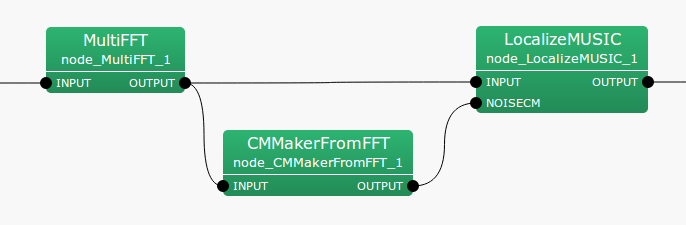## 6.2.4 CMMakerFromFFT

### 6.2.4.1 Module Overview

From the multi-channel complex spectrum that is output from the MultiFFT  node, generate the sound source correlation matrix with a fixed period.

None.

### 6.2.4.3 Usage

In what case is the node used?

Given a sound source of LocalizeMUSIC  node, in order to suppress a specific sound source like noise, etc., it is necessary to prepare a correlation matrix that includes noise information beforehand. This node generates the correlation matrix for a sound source, at fixed period, from a multi-channel complex spectrum that is output from the MultiFFT  node. Suppressed sound source can be achieved by connecting the output of this node to the NOISECM input terminal of LocalizeMUSIC  node, assuming that information before a fixed period is always noise.

Typical Examples

Figure. 6.20 shows the usage example of CMMakerFromFFT  node.

INPUT The input terminal is connected to the complex spectrum of the input signal calculated from a MultiFFT  node. The type is Matrix<complex<float> >  type. This node calculates and outputs the correlation matrix between channels for each frequency bin from the complex spectrum of an input signal. The output type is Matrix<complex<float> >  type, but to handle a correlation matrix, convert the three dimensional complex array to a two dimensional complex array and then output.Figure 6.20: Network Example using CMMakerFromFFT

### 6.2.4.4 I/O and property setting of the node

Table 6.22: Parameter list of CMMakerFromFFT
 Parameter Type Default Unit Description WINDOW 50 Number of averaged frames for a CM PERIOD 50 Frame rate for renewing the correlation matrix WINDOW_TYPE FUTURE Frame selection to normalize CM ENABLE_DEBUG false ON/OFF of debugging information output

Input

INPUT

: Matrix<complex<float> >  type, the complex spectrum expression of an input signal $M \times ( NFFT / 2 + 1)$.

Output

OUTPUT

: Matrix<complex<float> >  type. A correlation matrix for each frequency bin. An $M$-th order complex square array with correlation matrix outputs $NFFT/2 + 1$ items. Matrix<complex<float> >  indicates the rows corresponding to frequency ($NFFT/2 + 1$ rows), and columns containing the complex correlation matrix ($M * M$ columns across).

OPERATION_FLAG

: bool  type. This outputs trueif the correlation matrix from OUTPUT is updated. Otherwise false. This port is invisible by the default. To visualize it, see Fig. 6.33 in LocalizeMUSIC .

Parameter

WINDOW

: int  type. Default value is 50. Specifies the number of average smoothed frames when calculating the correlation-matrix. The node generates a correlation matrix for each frame from the complex spectrum of the input signal and outputs a new correlation matrix by averaging the frames that are specified in WINDOW. The correlation matrix calculated at the end is output between the PERIOD frames. If this value is increased, the correlation matrix is stabilized but the calculation cost becomes high.

PERIOD

: int  type. Default value is 50. Specifies the frame rate for renewing the correlation-matrix. The node generates a correlation matrix for each frame from the complex spectrum of the input signal and outputs a new correlation matrix by averaging the frames that are specified in WINDOW. The correlation matrix calculated at the end is output between the PERIOD frames. If this value is increased, the time resolution of correlation matrix is improved but the calculation cost becomes high.

WINDOW_TYPE

: string  type. FUTURE is the default value. The selection of used smoothing frames for correlation matrix calculation. Let $f$ be the current frame. If FUTURE, frames from $f$ to $f+WINDOW-1$ will be used for the normalization. If MIDDLW, frames from $f-(WINDOW/2)$ to $f+(WINDOW/2)+(WINDOW\% 2)-1$ will be used for the normalization. If PAST, frames from $f-WINDOW+1$ to $f$ will be used for the normalization.

ENABLE_DEBUG

: bool  type. Default value is false. When true, the frame number is output to the standard output while generating the correlation matrix.

### 6.2.4.5 Module Description

The complex spectrum of the input signal output from a MultiFFT  node is represented as follows.

 \begin{equation} \label{eq:CMMakerFromFFT_ X} {\boldsymbol X}(\omega ,f) = [X_1(\omega ,f), X_2(\omega ,f), X_3(\omega ,f), \cdots , X_ M(\omega ,f)]^ T \end{equation} (1)

Here, $\omega$ is the frequency bin number, $f$ is the frame number for use with HARK  , $M$ represents the number of input channels.

The correlation matrix of the input signal ${\boldsymbol X}(\omega ,f)$ can be defined as follows for every frequency and frame.

 \begin{equation} \label{eq:CMMakerFromFFT_ R} {\boldsymbol R}(\omega ,f) = {\boldsymbol X}(\omega ,f){\boldsymbol X}^*(\omega ,f) \end{equation} (2)

Here, $()^*$ denotes the complex conjugate transpose operator. There is no problem if this ${\boldsymbol R}(\omega ,f)$ is used as it is in subsequent processing, but practically, in order to obtain a stable correlation matrix in HARK  , it uses an average through time as shown below.

 \begin{equation} \label{eq:CMMakerFromFFT_ Rn} {\boldsymbol R}’(\omega ,f) = \frac{1}{{\rm WINDOW}}\sum _{i=W_ i}^{W_ f}{\boldsymbol R}(\omega ,f+i) \end{equation} (3)

The frames used for the averaging can be changed by WINDOW_TYPE. If WINDOW_TYPE=FUTURE, $W_ i = 0$ and $W_ f = {\rm WINDOW}-1$. If WINDOW_TYPE=MIDDLE, $W_ i = {\rm WINDOW}/2$ and $W_ f = {\rm WINDOW}/2+{\rm WINDOW}\% 2-1$. If WINDOW_TYPE=PAST, $W_ i = -{\rm WINDOW}+1$ and $W_ f = 0$.

${\boldsymbol R}’(\omega ,f)$ is output by every PERIOD frame from the OUTPUT terminal of CMMakerFromFFT  node.